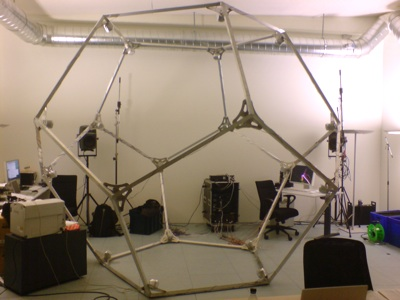Interactive Swarm Space

Spatialization Techniques

Multichannel Output

See Multichannel

Ambisonic Spatialization

### Introduction

Ambisonics is a surround-system for encoding and rendering a 3D sound field. While Ambisonic installations traditionally work with a Ambisonic encoder and a separate decoder, ISO's AEPUnit (Ambisonic Equivalent Panning Unit), combines these two into one single unit, that features a great advantage over traditional setups: The order of the ambisonic computation can be arbitrary, the performance is unaffected!! This has been discovered by none other then our project leader Martin Neukom and has been presented at AES 121st Convention, San Francisco. Kudos to you, Martin!

### AEPUnit in action

An AEPUnit represents a single-channel sound entity in space. Its 3D position can be controlled by a 3-channel ControlPort (x,y,z) and the aforementioned order is represented by a SwitchPort ("order"). When initializing an AEPUnit (i.e. calling an AEPUnit constructor), you need to specify the speaker positions of all your speakers, the more speakers you have, the better the spatial resolution. These measurements have to be precise, offsets of some centimeters can affect high frequencies which are very important for spatial hearing.

As an example here's how we setup the AEPUnit for our dodecahedron "ISOkaeder"

```QVector< math::Vector3 >speakerPos;
speakerPos.append( math::Vector3<float>( 0, 0.607062, -0.794654 ) );speakerPos.append( math::Vector3<float>( 0.57735, 0.187592, -0.794654 ) );speakerPos.append( math::Vector3<float>( 0.356822, -0.491123, -0.794654 ) );speakerPos.append( math::Vector3<float>( -0.356822, -0.491123, -0.794654 ) );speakerPos.append( math::Vector3<float>( -0.57735, 0.187592, -0.794654 ) );speakerPos.append( math::Vector3<float>( 0, 0.982247, -0.187592 ) );speakerPos.append( math::Vector3<float>( 0.934172, 0.303531, -0.187592 ) );speakerPos.append( math::Vector3<float>( 0.57735, -0.794654, -0.187592 ) );speakerPos.append( math::Vector3<float>( -0.57735, -0.794654, -0.187592 ) );speakerPos.append( math::Vector3<float>( -0.934172, 0.303531, -0.187592 ) );speakerPos.append( math::Vector3<float>( -0.57735, 0.794654, 0.187592 ) );speakerPos.append( math::Vector3<float>( 0.57735, 0.794654, 0.187592 ) );speakerPos.append( math::Vector3<float>( 0.934172, -0.303531, 0.187592 ) );speakerPos.append( math::Vector3<float>( 0, -0.982247, 0.187592 ) );speakerPos.append( math::Vector3<float>( -0.934172, -0.303531, 0.187592 ) );speakerPos.append( math::Vector3<float>( -0.356822, 0.491123, 0.794654 ) );speakerPos.append( math::Vector3<float>( 0.356822, 0.491123, 0.794654 ) );speakerPos.append( math::Vector3<float>( 0.57735, -0.187592, 0.794654 ) );speakerPos.append( math::Vector3<float>( 0, -0.607062, 0.794654 ) );speakerPos.append( math::Vector3<float>( -0.57735, -0.187592, 0.794654 ) );AEPUnit* multiAepUnit = new AEPUnit("myAmbisonicUnit", speakerPos);```20-sided ISOkaeder at the ICST

Now that we have setup the speakerposition vector and supplied it to our AEPUnit, all we need to do is to feed it realtime audio data (source signal) and control its position inside the ambisonic space (flight path). Both can be done in realtime! Simply feed a single-channel audio source to the AudioInputPort ( mySource->connect(myAEPUnit); ) and fiddle with the "position" ControlPort. You can either just set it:

`myAEPUnit->set("position", Frame(3, 0.0, 0.0, 0.0)); `

... or send an event to it (see Events section)

```Synth::get().eventManager().createEvent(2000.0,
myAEPUnit->controlPort("position"),
Frame(3, 0.0, 0.0, 0.0), 20.0); ```

... or connect it with a 3-channeled control data generator like this one:

```WaveTableOscil* pos_control = new WaveTableOscil(3, "sinewave");
pos_control->connect(myAEPUnit, "position");```

Or, and this is what Interactive Swarm Orchestra is all about, control it by ISOFlock:

`//todo`

... and these are only a few of the options you have when controlling your Ambisonic panning.